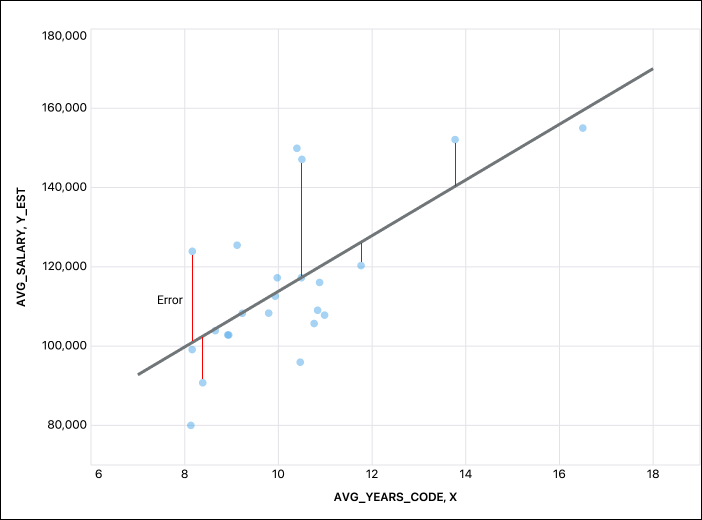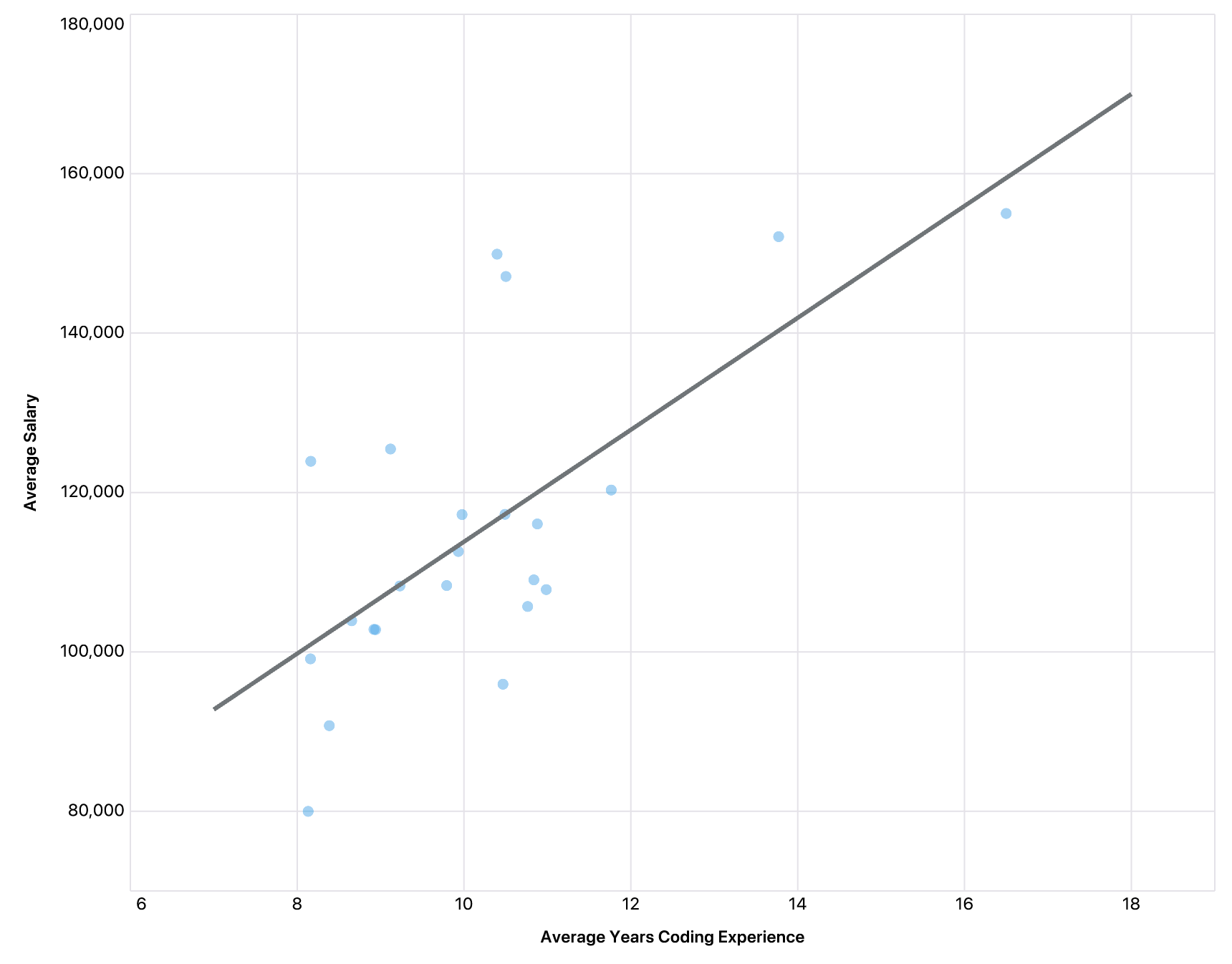SQL Resources/Snowflake/Linear Regression

# Linear Regression

Linear regression is a powerful way to understand your data and make predictions. Doing this in SQL has always been difficult, but Snowflake has a few built-in functions that simplify the process.

## Crash course

Linear regression is the process of fitting a linear model to observed data. This often takes the shape of fitting a trend line to data.

These lines can then be used to:

1. Better understand the relationship between the two variables
1. Make predictions about un-observed values

There are many variations and methodologies to calculate this trend line, but the most common is called Least-Squares Regression, which attempts to minimize, on average, the distance between the fitted line and the observed points.The idea is to place a line that minimizes the sum of the squared residuals, represented as the red lines above.

``MINIMIZE SUM(error_1^2, error_2^2, ... , error_n^2)``

#### Finding the line

The line is represented by:

``y_est = mx + b``

where:

• y_est - estimated y value
• m - slope
• x - x value
• b - y-intercept

We can estimate m and b using the following formulas:

``````m = sum((x - x_bar) / (y - y_bar)) / sum((x-x_bar)^2)
b = y_bar - (m * x_bar)``````

#### Goodness of fit

Once we use these formulas to have our line, we can evaluate how well it fits our data using a metric called R-squared.

In effect, R-squared measures how much of the variance can be explained by our fit line.

``R-squared = sum((y_est - y_bar)^2) / sum((y - y_bar)^2)``

This will evaluate to a number between 0 and 1, with 1 being a better fitting line.

## Step-By-Step

Thankfully, Snowflake has some built-in functions to make finding a trend line much easier. These include:

Let's see how to use them to make the following plot:``SELECT AVG_YEARS_CODE, AVG_SALARY, DEV_TYPE FROM PUBLIC.SO_SALARY``
AVG_YEARS_CODE
10.83692773
10.50219539
10.98557182
10.76276576
10.87868481
16.49919485
13.77134029
8.159303086
8.38508884
8.16277105
8.131296718
10.49096595
9.976124375
9.791820753
9.931633207
10.46674401
10.3957529
8.653050774
8.919727838
9.118639139
8.939187347
9.230899114
11.76445024
AVG_SALARY
109036.0829
147103.3569
107815.8756
105691.588
116052.123
155010.0265
152102.1363
99119.27968
90740.52521
123911.1833
79984.80214
117256.6903
117228.032
108321.9202
112595.8757
95941.5808
149901.0961
103901.0658
102814.5598
125454.2183
102792.3237
108271.3855
120308.0049
DEV_TYPE
Engineer; site reliability
Developer; desktop or enterprise applications
Developer; embedded applications or devices
Senior executive/VP
Engineering manager
Developer; front-end
Developer; mobile
Data scientist or machine learning specialist
DevOps specialist
Designer
Scientist
Educator
Marketing or sales professional
Developer; full-stack
Developer; back-end
Engineer; data
Developer; QA or test
Developer; game or graphics
Product manager

#### 1. Find The Slope and Intercepts

Using REGR_SLOPE and REGR_INTERCEPT, we can quickly find the estimated slope and intercepts:

``````SELECT
REGR_SLOPE(AVG_SALARY,AVG_YEARS_CODE) m,
regr_intercept(AVG_SALARY,AVG_YEARS_CODE) b
from PUBLIC.SO_SALARY``````
M
7022.130523674
B
43603.458640385

So now our line can be modeled with:

``y_est = 7022.131X + 43603.459``

#### 2. Generate values for the line

In order to add the line to the chart, we can generate x values for the trend line above. To do this we can use the GENERATOR function to generate rows throughout the range of the Years of Experience we have in our dataset.

``SELECT row_number() over (order by seq4()) + 6 x FROM table(generator(ROWCOUNT => 12))``
X
7
8
9
10
11
12
13
14
15
16
17
18

Then we can use our trend line equation to generate the Y values for our trend line:

``Select LINE_X.X, LINE_X.X * M + B y_est from LINE_X cross join SLOPES``
X
7
8
9
10
11
12
13
14
15
16
17
18
Y_EST
92758.372306104
99780.502829778
106802.633353452
113824.763877126
120846.8944008
127869.024924474
134891.155448148
141913.285971823
148935.416495497
155957.547019171
162979.677542845
170001.808066519

#### 3. Join trend line points back to original data

``Select * from A full outer join LINE_XY ON ROUND(A.AVG_YEARS_CODE) = LINE_XY.X``
AVG_YEARS_CODE
10.83692773
10.50219539
10.98557182
10.76276576
10.87868481
16.49919485
13.77134029
8.159303086
8.38508884
8.16277105
8.131296718
10.49096595
9.976124375
9.791820753
9.931633207
10.46674401
10.3957529
8.653050774
8.919727838
9.118639139
8.939187347
9.230899114
11.76445024
NULL
NULL
NULL
NULL
NULL
AVG_SALARY
109036.0829
147103.3569
107815.8756
105691.588
116052.123
155010.0265
152102.1363
99119.27968
90740.52521
123911.1833
79984.80214
117256.6903
117228.032
108321.9202
112595.8757
95941.5808
149901.0961
103901.0658
102814.5598
125454.2183
102792.3237
108271.3855
120308.0049
NULL
NULL
NULL
NULL
NULL
DEV_TYPE
Engineer; site reliability
Developer; desktop or enterprise applications
Developer; embedded applications or devices
Senior executive/VP
Engineering manager
Developer; front-end
Developer; mobile
Data scientist or machine learning specialist
DevOps specialist
Designer
Scientist
Educator
Marketing or sales professional
Developer; full-stack
Developer; back-end
Engineer; data
Developer; QA or test
Developer; game or graphics
Product manager
NULL
NULL
NULL
NULL
NULL
X
11
11
11
11
11
16
14
8
8
8
8
10
10
10
10
10
10
9
9
9
9
9
12
18
15
17
13
7
Y_EST
120846.8944008
120846.8944008
120846.8944008
120846.8944008
120846.8944008
155957.547019171
141913.285971823
99780.502829778
99780.502829778
99780.502829778
99780.502829778
113824.763877126
113824.763877126
113824.763877126
113824.763877126
113824.763877126
113824.763877126
106802.633353452
106802.633353452
106802.633353452
106802.633353452
106802.633353452
127869.024924474
170001.808066519
148935.416495497
162979.677542845
134891.155448148
92758.372306104

Now we have our scatter with our calculated trend line!

#### 4. Evaluate goodness of fit

Now we can evaluate how well our line fits our data using REGR_R2:

``SELECT regr_r2(AVG_YEARS_CODE,AVG_SALARY) R_2 FROM PUBLIC.SO_SALARY``
R_2
0.4651945324

An R-squared of 0.465 is not ideal, but it tells us how much we should (or shouldn't) trust the predictions we make with this equation.

#### 5. Make predictions!

Now we can use our formula to work out for <X> years of coding experience, what a developer's average salary may be:

``````SELECT 5 years_experience, SLOPES.M * 5 + SLOPES.B Estimated_salary FROM SLOPES
union all
(SELECT 15 years_experience, SLOPES.M * 15 + SLOPES.B Estimated_salary FROM SLOPES)``````
YEARS_EXPERIENCE
5
15
ESTIMATED_SALARY
78714.111258756
148935.416495497

### How We Built This

This page was built using Count, the first notebook built around SQL. It combines the best features of a SQL IDE, Data Visualization Tool, and Computational Notebooks. In the Count notebook, each cell acts like a CTE, meaning you can reference any other cell in your queries.

This makes not only for far more readable reports (like this one) but also a much faster and more powerful way to do your analysis, essentially turning your analysis into a connected graph of data frames rather than one-off convoluted queries and CSV files. And with a built-in visualization framework, you won't have to export your data to make your charts. Go from raw data to interactive report in one document.

Become a SQL ExpertGet five free SQL lessons from Count's Head of Data, Taylor Brownlow.
Email
Title